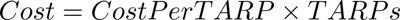Features

# Cost Per TARP Calculator

Cost Per Target Audience Rating Point or CPT is a significant metric in the marketing and advertising industries.

Target Audience Rating Point or TARPs are a key measure that has been used for many years to measure the effectiveness of above the line media campaigns. It provides a meausre of the percentage of the target audience viewing a given program at a given time. Cost per TARP or CPT is a measure of the cost per target audience rating point.

## Cost Per TARP Formulas

### Calculate CPT

To calculate cost per TARP simply dive the total costs by the total number of TARPs.### Calculate TARPs

To calculate the number of TARPS from the total cost and the cost per TARP, simply divide the total cost by the cost per TARP (CPT). Remember that if the CPT has been rounded in any way the number of TARPs may not be correct.### Calculate Cost

To calculate the total cost from the number of TARPs and the cost per TARP, simply multiply the cost per TARP by the number of TARPs.